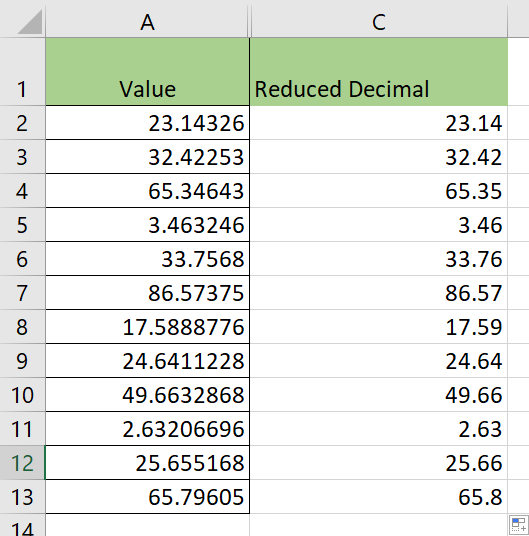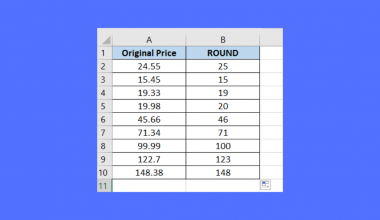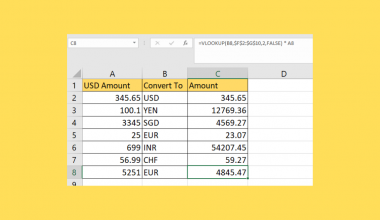# How to Reduce Decimals in Excel

This guide will explain several methods to reduce decimals in Excel.

Excel comes with several formatting options and functions that can be used to decrease the number of decimals in a specific numerical value.

This article will go over some common methods you can use to limit the number of decimal places of a particular value. But first, let’s look into a situation where you might need to reduce the number of decimals.

Suppose you are working on a financial sheet. Most currencies only support values up to the second decimal place. If a calculation in our worksheet returns a value of \$1.2002, we want to reduce the number of decimals to two and get \$1.20.

There are situations where you might want to remove decimals altogether and return a whole number. For example, it makes no sense to have a stock of 25.5 for a particular item in an inventory. The business owner can either round-up or round down the values to the nearest integer. How can we reduce the number of decimals in Excel?

First, we must explain the difference between formatting and truncating. Formatting cells so that less decimal places are seen does not change the actual value of the cell.

When we truncate or round a value, we are actually modifying the value of the decimal. It’s possible that reducing decimals in this manner will affect the accuracy of your sheet. Which method you should use depends on your use case.

Now that we know when to reduce decimals in Excel, let’s look at how these methods can be used on an actual sample spreadsheet.

## A Real Example of Reducing Decimals in Excel

Let’s take a look at a real example formatting and functions being used in an Excel spreadsheet to reduce decimals.

In the example below, we use cell formatting to control the number of decimal places shown in column C.In the second example, we used the `TRUNC` function to reduce the number of decimals in the value. The `TRUNC` function accepts up to two arguments: the number to truncate and the precision required. If the second argument is not provided, the `TRUNC` function will return an integer.To get the values in Column F, we just need to use the following formula:

`= TRUNC(A2, 2)`

If you’re ready to try out the methods we’ve mentioned in Excel, head over to the next section to read our step-by-step guide.

## How to Reduce Decimals in Excel

This section will guide you through each step needed to start reducing decimals in Excel. You’ll learn how we can use both cell formatting and mathematical functions to perform this task.

Follow these steps to start using cell formatting to reduce decimals:

1. First, select the cell or cell range to which you want to apply cell formatting. In this example, we’ll apply formatting to the values in column B. These values are a direct copy of the original values found in column A.2. Once your desired range is selected, go to the Home tab and look for the decrease decimals icon in the Number group. Keep clicking on the icon until you reach the desired number of decimals.In the above example, the values themselves do not change. If you want to mathematically truncate or round the value to a certain number of decimal places, we can use a few mathematical functions.

1. Use the `ROUND` function to return the target value rounded to the nearest number of digits. In the example below, we’ll use the `ROUND` function to return the value 23.14326 rounded to the nearest hundredths.2. Hit the Enter key to evaluate the function. In our example, the `ROUND` function has returned the value 23.14.3. We can drag down the formula to fill out the rest of the column.4. Alternatively, we can use either the `ROUNDDOWN` or `ROUNDUP` functions to perform our rounding. The `ROUNDDOWN` function will always round the value to the nearest decimal value lower than the current argument. The `ROUNDUP` function always rounds the value to the nearest decimal value higher than the current argument.5. We can use the `TRUNC` function to reduce decimals in Excel. Enter the value you want to truncate as the first argument. The second argument should indicate how many decimal places you want to keep in your result.1. What is the difference between TRUNC and INT?
The `TRUNC` and `INT` functions round down the number to an integer by default. The main difference is that the `TRUNC` function includes an optional argument that allows the user to determine the precision of the truncation.

These two functions also treat negative numbers differently. `TRUNC(-2.5)` returns -2 while `INT(-2.5)` returns -3 because -3 is the value when rounded down.

This step-by-step guide should be all you need to begin reducing decimals yourself in Excel. Our guide shows how easy it is to decrease the number of decimals in a value by applying cell formatting or using functions such as `TRUNC` or `ROUND`.

Reducing decimals is just one example of a mathematical task you can perform on your data in Excel. With so many other Excel functions available, you can surely find one that suits your use case.

Are you interested in learning more about what Excel can do? Subscribe to our newsletter to find out about the latest Excel guides and tutorials from us.### Get emails from us about Excel.

Our goal this year is to create lots of rich, bite-sized tutorials for Excel users like you. If you liked this one, you'd love what we are working on! Readers receive ✨ early access ✨ to new content.

##### You May Also Like## How to Sort IP Address from Low to High in Excel

This guide will explain in detail how you can sort IP addresses from low to high in Microsoft…## How to Populate a Combo Box with Unique Values in Excel

This guide will explain how you can populate a combo box with unique values only in Excel. Adding…## How to Remove Decimals with Rounding in Excel

This guide will show and compare several Excel functions that we can use to remove decimals with rounding.…## How to Recover an Unsaved Document in Excel

This guide will show you how you can recover an unsaved Excel file in your computer. There may…